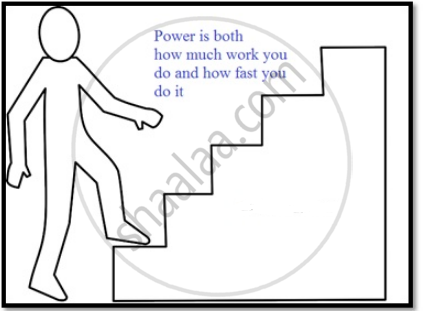Share

# Power

## Power

• Power is defined as the time rate at which work is done or energy is transferred.Average Power:
Ratio of work done(W) in a total time interval of t.
P_(av)= W/t

Instantaneous Power:
When the time interval t, approaches zero the limiting value of average power becomes instantaneous power.
P = (dW)/dt

• We can write W = F. dr,
P = F . (dr)/dt
P = F.v , where v is instantaneous velocity.

• Power is a scalar quantity

• SI unit of power – Watt (W)

• Its dimensions are [ML2T–3].

• 1 hp = 746 W

• We encounter the unit watt when we buy electrical goods such as bulbs, heaters and refrigerators. A 100 watt bulb which is on for 10 hours uses 1 kilowatt hour (kWh) of energy.

100 (watt) × 10 (hour) = 1000 watt hour

= 1 kilowatt hour (kWh)

= 103 (W) × 3600 (s)

= 3.6 × 106 J

• Our electricity bills carry the energy consumption in units of kWh. Note that kWh is a unit of energy and not of power.

Example: A pump on the ground floor of a building can pump up water to fill a tank of volume 30 m3 in 15 min. If the tank is 40 m above the ground, and the efficiency of the pump is 30%, how much electric power is consumed by the pump?
Solution: P = W/t = (mgh)/t
mass of water to be moved = ρV = 30000 kg
g = 9.8
h = 40 m
t = 15 x 60 = 900 s
P = 13066.67 W = 13.067 kW
Now η = P/(P_(ACTUAL)) => P_(ACTUAL) = 13.067/0.3 = 43.6 kW

S